# How to Make Money Trading the Harmonic Price Patterns in Olymp Trade.

Visit Website
Features
Bonus
Review
Register
• Demo Account
• :Regulated
• :MT4 Integration
• :Supports All Devices
• :Fast Payouts
• :Instant Deposits
100% Bonus for Deposits Made Within the Hour of Registration
* * Risk Warning: Forex and FTT trading involve significant risk to your invested capital.العربيةবাংলা简体中文繁體中文EnglishFilipinoગુજરાતીहिन्दीBahasa Indonesia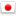日本語Bahasa MelayuਪੰਜਾਬੀСрпски језикسنڌيසිංහල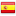Españolதமிழ்ไทยTürkçeاردو

Trends are harmonic in nature such that they can be broken into smaller or larger waves which can be used to predict future prices. But what really goes into finding harmonic price patterns in Olymp Trade, let’s find out.

What are Harmonic Price Patterns?

Harmonic price patterns are patterns in price movement which resemble geometric constructions and the application of Fibonacci numbers on them can be used to predict future price movements.

Trends are harmonic in nature such that they can be broken into smaller or larger waves which can be used to predict the price movements. These useful waves are the ones that take the form of specific geometric constructions.

However, the geometric patterns are useless if Fibonacci numbers are not applied to them.

Having said that, it is now clear that;

• A specific pattern of price movements will have to be waited for in order to develop.
• After that, Fibonacci numbers will be applied to the pattern to confirm if it is a valid or invalid signal.

The reason we emphasize on the Fibonacci numbers is that if they do not favor a certain price pattern, then the whole pattern is invalid and unworthy of trading. Even if the price forms into the very exact form of a pattern that is favored by the Fibonacci numbers, if Fibonacci does not confirm the signal then the whole thing would be invalid.

Essentially, Harmonic patterns provide accurate points where the price is bound to Reverse by use of the patterns coupled with Fibonacci ratios.

## But what really are these Fibonacci Numbers and Ratios?

Fibonacci numbers are key numbers used to create technical indicators. They were first identified by Leonardo Fibonacci in the 13th Century.

These numbers occur in a sequence beginning with 0 and 1 and the subsequent numbers are achieved by adding the two numbers prior to it.

Visit Website
Features
Bonus
Review
Register
• Demo Account
• :Regulated
• :MT4 Integration
• :Supports All Devices
• :Fast Payouts
• :Instant Deposits
100% Bonus for Deposits Made Within the Hour of Registration
* * Risk Warning: Forex and FTT trading involve significant risk to your invested capital.
• Regulated
• :Available on all devices
• :Demo Account Available
Free Demo Account
* * Risk Warning: Forex and CFD trading involves significant risk to your invested capital.
• Over 100 assets
• :Technical analysis tools
• :Fast and efficient on any device
100% Bonus for Deposits Made Within the Hour of Registration
* * Risk Warning: Options trading involves significant risk to your invested capital.

So the sequence would be something like 0,1,(0+1)=1,(1+1)=2,(1+2)=3,(3+2)=5,(3+5)=8,(5+8)=13,21,34,55,89,144…and so on.

One significant feature of these numbers is that each is approximately 1.618 times greater than the number before it. That striking feature is the basis on which the Fibonacci ratios are founded.

Fibonacci ratios are specific significant ratios derived from Fibonacci numbers. Fibonacci ratios find wide application in technical analysis to spot retracement levels.

The key Fibonacci ratios are 61.8%, 38.2% and 23.6%. 100% and 50% which have also found wide use by traders.

61.8% is achieved by dividing a Fibonacci number by the number immediately after it to the right of the sequence. For example, 13 divided by 21 gives 0.6190 while 89 divided by 144 gives 0.6180.

38.2% is achieved by dividing a Fibonacci number by the number in the second spot after it to the right of the sequence. For example, 13 divided by 34 gives 0.3824 while 55 divided by 144 gives 0.3819.

23.6% is derived from dividing a Fibonacci number by the number in the third spot after it to the right of the sequence. For example, 8 divided by 34 gives 0.2353 while 21 divided by 89 gives 0.2360.

To trade using the Harmonic price patterns, realize that the primary Fibonacci ratio is 0.618 or it’s derivative like 1.618. Complementing Fibonacci ratios to the primary include 0.382, 0.50, 1.41, 2.0, 2.24, 2.618, 3.14 and 3.618 derived from Fibonacci numbers as explained above. Realize also that these price patterns repeat themselves and you will be well suited to begin trading.

Harmonic patterns occur in various types. Each of these patterns displays a different geometric construction from the others and thus the difference. The variety includes 4 patterns as follows;

1. The Gartley.
2. Butterfly.
3. The Bat.
4. Crab.

Each of these patterns has both a bearish and a bullish pattern. The bearish pattern will give a Sell signal as it hints a reversal downwards while the Bullish pattern will give a Buy signal as it points towards a reversal upwards.

Also, each of these patterns will be explained with price moving progressively from point Q to A, B, C, and finally, D. Pay keen attention.

## 1. The Gartley.

Bullish pattern – This pattern will signify that an upward trend is about to set in. A Buy signal is thus generated at the completion of the pattern.

The price will move from a point Q upwards to Point A then a price correction downwards to point B. The price will then move upwards to C and then a retracement will occur downwards to the final point D. The point D will still be at a level above the point Q where the price movement originated.Bearish pattern – This pattern, contrary to the bullish one, will show that a downward move is imminent. That way, a Sell signal is generated at the end of the pattern.

Here, the price will move from a point Q downwards to point A followed by a retracement upwards to point B. The price will then move downwards to point C then an upward retracement to the final point D. The point D will still be below the point Q where the movement originated.

Now, those price movements alone are not enough proof that after the price will reverse upwards or downwards. You now bring the Fibonacci ratios in to determine whether it is a valid or invalid signal.

The measurements will exactly be the same for every wave across the Bullish and Bearish patterns. They will be as follows;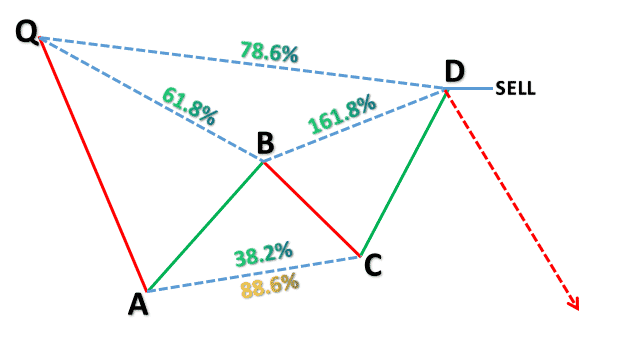Once these conditions of measurements are met, observe a reversal upwards at point D for the bullish pattern, and then enter a Buy position. Observe a reversal downwards at point D for the bearish pattern and enter a Sell position. Place your stop loss just below the point D for a Buy and just above the point D for a Sell.

## 2. The Butterfly.

Bullish pattern – This pattern will generate a Buy Signal because it will signify a price reversal upwards at its completion.

The Price will move from a point Q upwards to point A then a retracement downwards to the point B. This will be followed by a price movement upwards to the point C then a retracement downwards to the final point D. The point D will be at a level further below the point Q where the price movement began.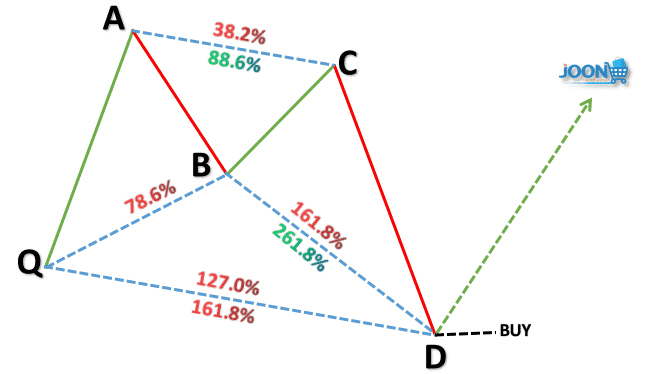Bearish pattern – This pattern will generate a Sell signal as it signifies a price reversal downwards at its completion.

Here, the price will move from a point Q downwards to point A then a retracement upwards to point B. The price will then move downwards to point C then a retracement upwards to the final point D. The point D will be at a level further above the point Q where the price movement began.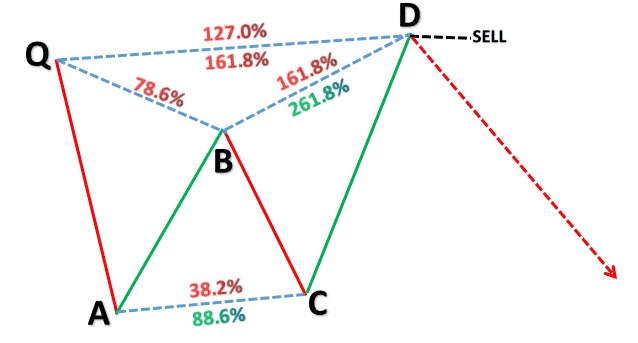If measurement conditions shown on the image above are met for a bullish pattern, observe a price reversal upwards at the point D and the Buy. If they are met for a bearish pattern, observe a reversal downwards at the point D then enter a Sell position. Your Stop loss is just below point D for a Buy and just above for a Sell.

## 3. The Bat.

Bullish BAT pattern – This pattern will generate a Buy Signal because it will signify a price reversal upwards at its completion.

The price will rise from the point Q to the point A then retrace downwards to B. The price moves up to C then retraces again downwards to the final point D. The point D, like in the Gartley pattern, will still be at a level above point Q where the price movement originated.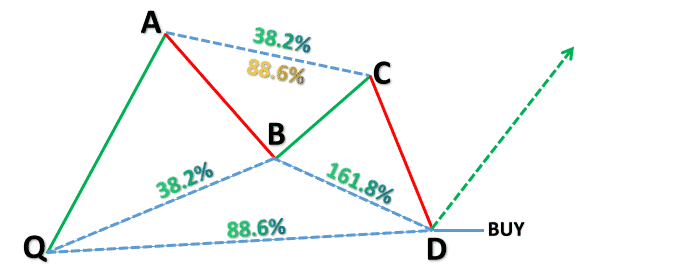Bearish BAT pattern – This pattern will generate a Sell signal as it signifies a price reversal downwards at its completion.

The price will fall from point Q to point A then retrace upwards to B. The price then moves down to C followed by a retracement upwards to the final point D. The point D, like in the Gartley pattern, will still be at a level below point Q where the price movement originated.Wait for either buy or sell conditions to be met depending on the pattern type – bullish or bearish. After they are met, observe reversal at point D in order to enter a Buy position for an upward reversal and a Sell for a downward reversal. Remember to place you stop loss close to the point of entry.

## 4. The Crab.

Bullish pattern – This pattern will generate a Buy Signal because it will signify a price reversal upwards at its completion.

The Price will move from a point Q upwards to point A then a retracement downwards to point B. This will be followed by a price movement upwards to the point C then a retracement downwards to the final point D. The point D, just like in the Butterfly, will be at a level further below the point Q where the price movement began.Bearish pattern – This pattern will generate a Sell signal as it signifies a price reversal downwards at its completion.

Here, the price will move from a point Q downwards to point A then a retracement upwards to point B. The price will then move downwards to point C then a retracement upwards to the final point D. The point D, just like in the Butterfly, will be at a level further above the point Q where the price movement began.Once the conditions are met, enter the right positions placing the Stop loss just above and just below the point D for quick exit in case of fake outs. You know that you need to wait for the reversal to occur before you enter a Buy for an upward reversal and a Sell for a downward reversal.

## Wrapping Up on Harmonic Patterns.

Just as we mentioned at the beginning, price movements must form patterns which take the form of geometric constructions. Fibonacci ratios then come into play to validate or invalidate the constructions. You clearly have seen how that happens as we have discussed in detail above.

Harmonic patterns are reversal patterns as we have seen. At the completion of each type, the price must reverse and take a different direction from before. Traders take advantage of this to profit big time from such reversals which have very high chances of occurring.

Take advantage of these accurate patterns and apply them to your Olymp Trade charts today to see the difference.

Visit Website
Features
Bonus
Review
Register
• Demo Account
• :Regulated
• :MT4 Integration
• :Supports All Devices
• :Fast Payouts
• :Instant Deposits
100% Bonus for Deposits Made Within the Hour of Registration
* * Risk Warning: Forex and FTT trading involve significant risk to your invested capital.

*Risk warning:

The information provided does not constitute a recommendation to carry out transactions. When using this information, you are solely responsible for your decisions and assume all risks associated with the financial result of such transactions.العربيةবাংলা简体中文繁體中文EnglishFilipinoગુજરાતીहिन्दीBahasa Indonesia日本語Bahasa MelayuਪੰਜਾਬੀСрпски језикسنڌيසිංහලEspañolதமிழ்ไทยTürkçeاردو